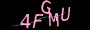×

# 报错信息来了：SyntaxError: Malformed formal parameter### 示例

#### 无效的

```var f = Function("x y", "return x + y;");
// SyntaxError (missing a comma)

var f = Function("x,", "return x;");
// SyntaxError (extraneous comma)

// SyntaxError (numbers can't be argument names)```

#### 有效的

```var f = Function("x, y", "return x + y;");  // correctly punctuated

var f = Function("x", "return x;");

// if you can, avoid using Function - this is much faster
var f = function (x) { return x; };```

https://www.jiangweishan.com/article/error20210602a3.html

### 网友评论文明上网理性发言已有0人参与